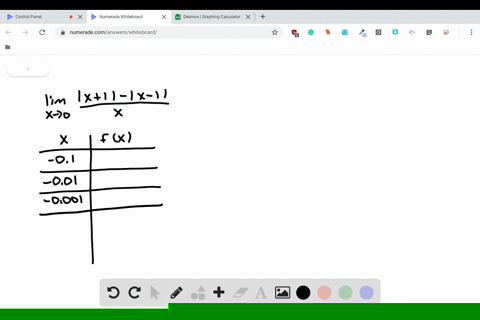Enroll in one of our FREE online STEM bootcamps. Join today and start acing your classes!View Bootcamps### by evaluating $f$ at $x$ -values near $0 .$ Sketc…

01:22Westview High School
Problem 69

# Estimating a Limit Consider the function$f(x)=(1+x)^{1 / x}$Estimate$$\lim _{x \rightarrow 0}(1+x)^{1 / x}$$by evaluating $f$ at $x$ -values near $0 .$ Sketch the graph of $f$

## Discussion

You must be signed in to discuss.

## Video Transcript

we're given the following limit as X approaches zero of the function one plus x two, the one over X power. And so we're asked to estimate this limit, Couldn't you waste so first of all, we are asked to created table to estimate it. So we have sex and effort backs and we know that approaches zero. So we need to create a values closer to zero. So we have neighbors 0.1 actual serving a good one negative. 0.1 No, ive is 0.1 Then we also do possible this around it. So we have 0.1 0.10 point one. And now the best way to estimate this is really just depressed. We figure this out is a punk thes rallies and your calculator. So you will get approximately achieve point six for Sorry. Two point 868 2.719 2.718 and then 2.716 She points 705 and she went for 94 Now, based off from this information, you can see that this is a spot where so This is a spot where zero is between and it seems to be approaching chill 0.717 So you can say that this limit is approximately 2.717 We want to check this race off photograph, so we graft dysfunction. And if we zoom in zero and we zoom in closer, we can see that it seems to be approaching around. There seems to be approaching 2.717 once again. So based off with this but based off of both our graph in her table when you say that limit as X approaches era of one plus x 2 to 1 over X power, it's approximately 2.717